﻿ Application of Artificial Neural Networks in Cold Rolling ProcessInternational Journal of Control Science and Engineering

p-ISSN: 2168-4952    e-ISSN: 2168-4960

2018;  8(1): 22-30

doi:10.5923/j.control.20180801.03### Application of Artificial Neural Networks in Cold Rolling Process

Tamaraebi Atuwo

Lamar University, Beaumont, US

Correspondence to: Tamaraebi Atuwo, Lamar University, Beaumont, US.
 Email: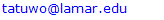Abstract

Rolling is one of the most complicated processes in metal forming. Knowing the exact amount of basic parameters, especially inter-stand tensions can be effective in controlling other parameters in this process. Inter-stand tensions affect rolling pressure, rolling force, forward and backward slips and neutral angle. Calculating this effect is an important step in continuous rolling design and control. Since inter-stand tensions cannot be calculated analytically, attempt is made to describes an approach based on artificial neural network (ANN) in order to identify the applied parameters in a cold tandem rolling mill. Due to the limited experimental data, in this subject a five stand tandem cold rolling mill is simulated through finite element method. The outputs of the FE simulation are applied in training the network and then, the network is employed for prediction of tensions in a tandem cold rolling mill. Here, after changing and checking the different designs of the network, the 11-42-4 structure by one hidden layer is selected as the best network. The verification factor of ANN results according to experimental data are over R=0.9586 for training and testing the data sets. The experimental results obtained from the five stands tandem cold rolling mill. This paper proposed new ANN for prediction of inter-stand tensions. Also, this ANN method shows a fuzzy control algorithm for investigating the effect of front and back tensions on reducing the thickness deviations of hot rolled steel strips. The average of the training and testing data sets is mentioned 0.9586. It means they have variable values which are discussed in details in section 4. According to Table 7, this proposed ANN model has the correlation coefficients of 0.9586, 0.9798, 0.9762 and 0.9742, respectively for training data sets and 0.9905, 0.9798, 0.9762 and 0.9803, respectively for the testing data sets. These obtained numbers indicate the acceptable accuracy of the ANN method in predicting the inter-stand tensions of the rolling tandem mill. This method provides a highly accurate solution with reduced computational time and is suitable for on-line control or optimization in tandem cold rolling mills. Due to the limited experimental data, for data extraction for the ANN simulation, a 2D tandem cold rolling process is simulated using ABAQUS 6.9 software. For designing a network for this rolling problem, various structures of neural networks are studied in MATLAB 7.8 software.

Keywords: Artificial neural networks, Computational time, On-line control, Finite element modeling, Training and testing data, Tandem cold rolling mill, Hidden layer

Cite this paper: Tamaraebi Atuwo, Application of Artificial Neural Networks in Cold Rolling Process, International Journal of Control Science and Engineering, Vol. 8 No. 1, 2018, pp. 22-30. doi: 10.5923/j.control.20180801.03.

### 1. Introduction

In almost all manufacturing industries, the rolling robots is one of the most common and complicated processes . Among all kinds of the rolling processes, the flat rolling is the most practical one. Many studies are conducted to enhance the quality and quantity of rolling products by optimizing and adaptive controlling the parameters . The mathematical modelling of the rolling process involves numerous parameters that would lead to nonlinear equations. This mathematical model needs significant computational time, which prevents its application in on-line control and supervision systems. Therefore, new and efficient methods are necessary to represent this kind of process .
The analysis of the rolling process is a high concern among many researchers with respect to the complexity of the process. There exist various applicable methods for studying the treatment of the rolling process. The finite element method (FEM) and slab method are the most common analytical methods often used for simulation [4-12]. All of these methods could reasonably predict the distribution of different parameters such as stress, strain, deformation and temperature [13, 14]. Among the different available techniques, (FEM) is preferred; as it can incorporate non-homogeneity of deformation, strain hardening, different friction models and many other real-life phenomena .
Although (FEM) is a very powerful tool for simulation of the engineering problems, the FE simulation of nonlinear problems is a time-consuming procedure. Nowadays, steel and aluminum manufacturing industries resort to online fault detection in the rolling process with the objective to eliminate the faults during the manufacturing process. To achieve this objective, faster prediction methods for predicting the behaviour of the slab under rolling are needed. An excellent solution to this problem is the application of the artificial neural networks (ANNs). The (ANN) has received a great importance in the last decade, due to its capability in solving non-linear problems [16-21]. For this purpose, training the designed network through the experimental data or the FE simulation results is necessary [22, 23].
The (ANN) is an important applicable tool in all of the scientific fields. Nowadays this method is adopted in many engineering applications especially rolling process. Ghaisari et al. (2012) proposed the application of (ANN) in predicting the internal mechanical properties of cold rolling products. In his study, the inputs into the proposed intelligent system included a wide variety of parameters used to predict the mechanical properties such as Yield Strength (YS), Ultimate Tensile Strength (UTS), and Elongation (EL) as the outputs. Results indicate that the thickness reduction in tandem affects the YS and EL values significantly, but the (UTS) is not sensitive to this parameter . Lee et al. (2004) proposed a long-term learning method applying (ANN) to improve the accuracy of rolling force prediction in a hot rolling mill. The statistical analysis showed that the overall thickness accuracy at the first coil of the lot was very low compared to that of the other coils. By doing so, the thickness error at the head-end part of the strip is reduced considerably . Bagheripoor and Bisadi (2013) introduced an (ANN) application to a hot strip mill to improve the model’s prediction ability for rolling force and rolling torque. In their study, numerous three dimensional finite element simulations were carried out in order to obtain a data basis for training and validation of the neural network. The resulted ANN model is feasible for on-line control and rolling schedule optimization [26-28].
There exist some studies in determining the inter-stand tensions in rolling process, but because simplified assumptions were adopted in their works, achieving acceptable tensions values become impossible. The Dixit study is a sample of this phenomenon. In his work he estimated that the maximum safe level of the tension to reduce the possibility of tearing is about one-third of the yield stress . Among all the conducted studies, very few have adopted the (ANN) for prediction of inter-stand tensions in the recent past. Developed a fuzzy control algorithm for investigating the effect of front and back tensions on reducing the thickness deviations of hot rolled steel strips through (ANN). The results obtained in his study can be applied in establishing a database for Loop control in hot rolling processes by determining the effect of the front and back tensions and selected process parameters on maintaining the uniformity of the strip thickness . In other study, Arinton et al. (2012) introduced a multilayered approach of the high-order neural network that could be applied in a robust fault detection scheme. It is shown that these networks can approximate any function with less parameters than that of the multi-layer perceptron neural network. The application of these networks is for system identification and robust default detection of the inter-stand strip tension for a continuous five stands cold mill .
The objective of this study is an attempt to develop a neural network model for a tandem cold rolling mill capable of predicting accurate rolling tensions under various rolling conditions. A 2D (FEM) is developed for calculating the rolling parameters and training the network. The simulation results are validated using the experimental results obtained from the five stands tandem cold rolling mill [32, 33].
For simulation of this process, 11 parameters are considered as input parameters in the network. Two of these parameters are selected as the input and output thickness of the sheet, four as reductions and five as angular velocities of the rollers. The target output data are four inter-stand tensions. After training, the network can predict the response for the new applied data. The performance of the network is studied and indicates the promising application for online prediction and optimization in Hardware In the Loop (HIL) tests [34, 35].

### 2. FEM

Due to the limited experimental data, for data extraction for the ANN simulation, a 2D tandem cold rolling process is simulated using ABAQUS 6.9 software. Here, the effect of different process parameters such as the initial thickness of the strip, thickness reductions and rollers' speeds are studied and then the effect of these parameters on the inter-stand tensions is investigated using HIL simulation .

#### 2.1. Finite Element Modelling

The models of the parts for simulating the rolling process are constructed using the same design used in the experiments. The used experimental rolling conditions are summarized in table 1.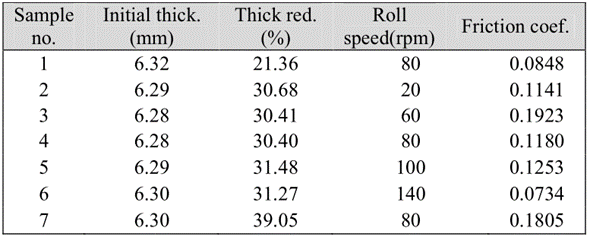Table 1. Condition of the tandem cold rolling experiments employed for the simulation
In this simulation, the rollers are assumed to be rigid and the sheet is discretized into 400 elements. Because of inconsiderable changes in the width of the sheet during rolling, this process is considered as plane strain and a 2D simulation is implemented .
Here, the process is considered as axisymmetric and due to the symmetric nature of flat rolling process, only one half of the strip is modeled. This model consists of 4 node quadrilateral shell elements. The rolling is performed in a 5-stand mill with roll diameters of 520.1, 541.2, 566.7, 574.3 and 584.6 mm, respectively. The relevant properties of the used st1521 sheet in the simulation are expressed in Table 2. Here, the flow rule is expressed by simple power law: where K is the strength coefficient and n is Strain hardening exponent. The material properties for st1521 sheet is obtained from the tensile test and is considered as k=640 Mpa, n=0.184.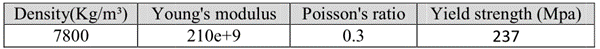Table 2. Mechanical properties of st1521 sheet
For simulation, the Coulomb friction model is assumed in this study.
The changing contour of tension during simulation is shown in Figure 1. As expected the rolling tensions gap and reach relatively steady values when the deformation is in the steady state region.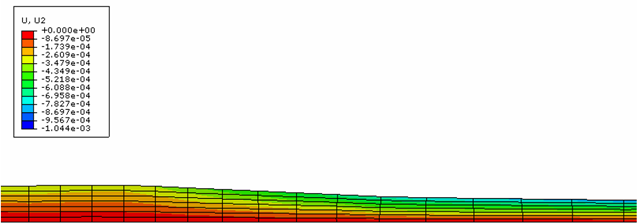Figure 1. Changing the contour of tension in the first

#### 2.2. Model Verification

The FEM model developed in this investigation is validated by comparing the model predictions of inter-stand tensions stand tandem mill. A comparison among the FEM predicted values of inter-stand tensions with experimental data is presented in Figure 2. As observed, the prediction of relative error has a mean value of 9.2% for inter the tensions. A good correlation between the numerical and experimental results is observed in Figure 2. So, the FE model is able to produce accurate tensions data for the rolling process under investigation, and can be used to generate the training data for ANN modeling.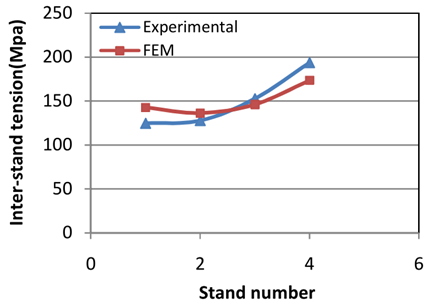Figure 2. Comparison among the FEM predicted values and experimental data

### 3. Artificial Neural Network (ANN)

In the last decade, the (ANN) is being proposed as a powerful computational tool due to its low time consumption in processing. The most common applications for (ANN) are function approximation, pattern recognition and classification . A standard (ANN), depicting its most important parts: the synapses, characterized by their weight values, the summing junction (integrator), and the activation function is illustrated in Fig. 3. An input signal at the input of synapse j connected to neuron k is multiplied by the synaptic weight. The summing junction adds all the input signals weighted by the synaptic weight values plus the neurons’ bias. This operation constitutes the dot (or inner) product among the inputs and the weight values, plus the bias. Finally, an activation function is used to limit the amplitude of the output of the neuron. The output of neuron k can be described by a simple equation mathematically: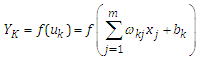(1)
Where, j = 1,..., m, are the input signals, j = 1,..., m, are the synaptic weights of neuron k, is the net input to the activation function, is the neuron bias, f (·) is the activation function and is the output. The activation function, denoted by, determines the output of a neuron k in relation to its network input. This function can assume a number of forms most frequently used ones are the linear function, the threshold function, the radial basis functions (e.g., Gaussian function), and the sigmoid functions (e.g., logistic and hyperbolic tangent).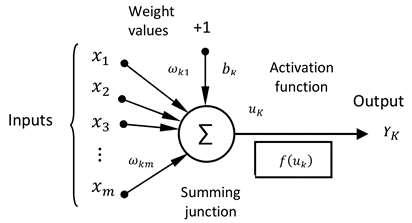Figure 3. Nonlinear model of a neuron

#### 3.1. Neural Network Design

Neural networks consist of parallel processing architectures where various nodes called neurons are interconnected . These can be used for finding the correlation between independent and dependent variables in complicated problems. Although there are different types of networks that can be used for finding the correlation between the input and output parameters, all of them have a unique purpose that is minimizing the network errors . Accuracy and efficiency of the neural networks depend on various parameters, such as number of patterns used in training and testing, number of input and output parameters, learning rate, number of hidden layers and number of neurons in the hidden layer. There is no exact formula available to decide which architecture of ANN and which training algorithm will solve a given problem. Some of the researchers determine these parameters by numerical experiments. In this study, the best solution is obtained by trial and error. For designing a network for this rolling problem, various structures of neural networks are studied in MATLAB 7.8 software.
Among possible network structures, single layer neural networks are not suitable. Because of complexity of rolling process, multilayer neural networks, along with various learning rules and structures, are examined. A general flowchart of this proposed ANN model is shown in Figure 4. It was draw again with Visio software.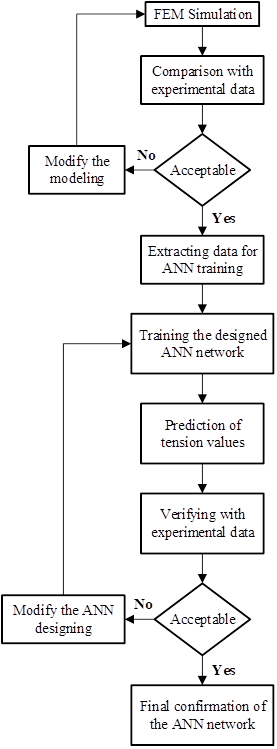Figure 4. General flowchart of the newly introduced ANN simulation method
In this study, a feed-forward artificial neural network containing a back propagation algorithm is applied, which are the most commonly applied ANN for modeling due to their powerful capabilities. The definition of the net structure, as the number of hidden layers and the number of neurons in those layers is not established yet. Although one layer with non-linear functional correlation is sufficient to map any non-linear functional relationship with a reasonable level of accuracy , sometimes more than one hidden layer should be employed . Here, the best architecture of the network is obtained through trying the different numbers of hidden layers and neurons. The trial begins with one hidden layer with many different network models were tried and the R values of the model are calculated. The type of learning applied here is known as the “supervised learning” and the training algorithm type applied is the “back-propagation”. The supervised learning of the ANN uses a training set for the input and output of the net. The two widely used training functions are Logsig and Tansig. Here, the Log-sigmoid function was chosen as the axon transfer function as it is the most consistent with the biophysics of the biological neuron. For the output layer, the used function is Purelin.
Among possible network structures, single layer neural networks are not suitable. Because of complexity of rolling process, multilayer neural networks, along with various learning rules and structures, are examined. A general flowchart of this proposed ANN model is shown in Figure 4.
According to the obtained results, in this work one hidden layer is considered for the simulated network. The neural networks considered in this study have 11 inputs, where the number of neurons in the output layers are chosen as 4, corresponding to the number of outputs of the nets. Based on this analysis, the optimal architecture of the ANN was constructed as 11–42–4, representing the total number of inputs, neurons in the hidden layer, and outputs, respectively. The applied (ANN) architecture and its functions are tabulated in Table 3. The type of learning applied here is known as the supervised learning and the training algorithm type applied is the “back-propagation”. The supervised learning of the ANN uses a training set for the input and output of the net. For each data set, the weights of the net are adjusted to minimize the error in the outputs of the net. The error is minimized using the gradient technique with a convergence factor named “learning rate”. The two widely used training functions are logsig and tansig . Here, the log-sigmoid function was chosen as the axon transfer function as it is the most consistent with the biophysics of the biological neuron. For the output layer, the used function is purelin [45, 46].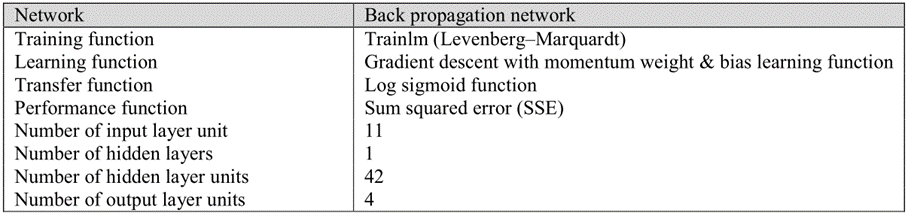Table 3. The ANN architecture and functions

#### 3.2. Input and Output Parameters

The neural network program is developed to construct a quantitative model for finding the inter-stand tensions in a tandem cold rolling mill. A 5-stand tandem cold rolling mill is illustrated in Figure 5.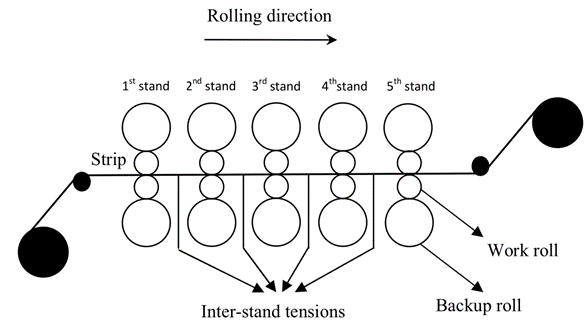Figure 5. Schematic Illustration of a 5-stand tandem mill
By selecting the most important parameters in this process, the 11 independent input parameters are selected as: input and output thickness of the sheet, four reductions and five angular velocities of the rollers. The target output parameters are the four inter-stand tensions. The input and output parameters of the ANN are listed in Table 4. The number of neurons in each layer of the ANN is selected as 11, 42 and 4. The used patterns of the neural network model are shown in Figure 6. The number of neurons in the hidden layer is obtained by trial and error. Once the network is trained to achieve the acceptable error, the weights and biases are fixed in model and the model is ready to be validated through the unseen dataset, i.e., the test data, not used for training for this purpose.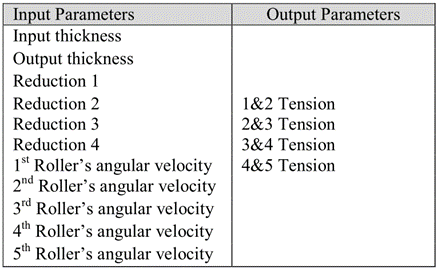Table 4. Input & output parameters of ANN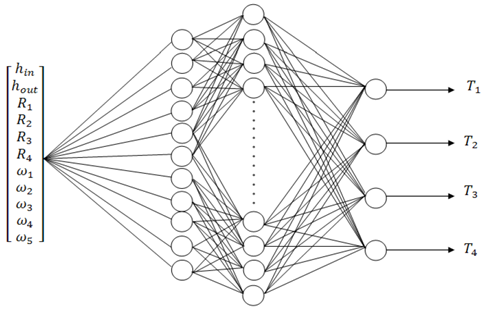Figure 6. Schematic illustration of the applied ANN network

#### 3.3. Training and Testing the Network

For training the network, enough input–output data are needed. Depending on the complexity of the simulated process, the required data for testing the network is different. For this reason, the initial values of the process parameters such as the input and output thicknesses, rolling speeds (AV) and thickness reductions (R) are extracted under different conditions, shown in Table 5 and 6, respectively for training and testing the data sets. For extracting these data, the (FEM) is used in ABAQUS 6.9 software. For this network, 60 sets of data (900 data) are extracted and applied for training the ANN with various process parameters. For testing this network, 10 sets of data (1050 data) are extracted and applied for testing the network.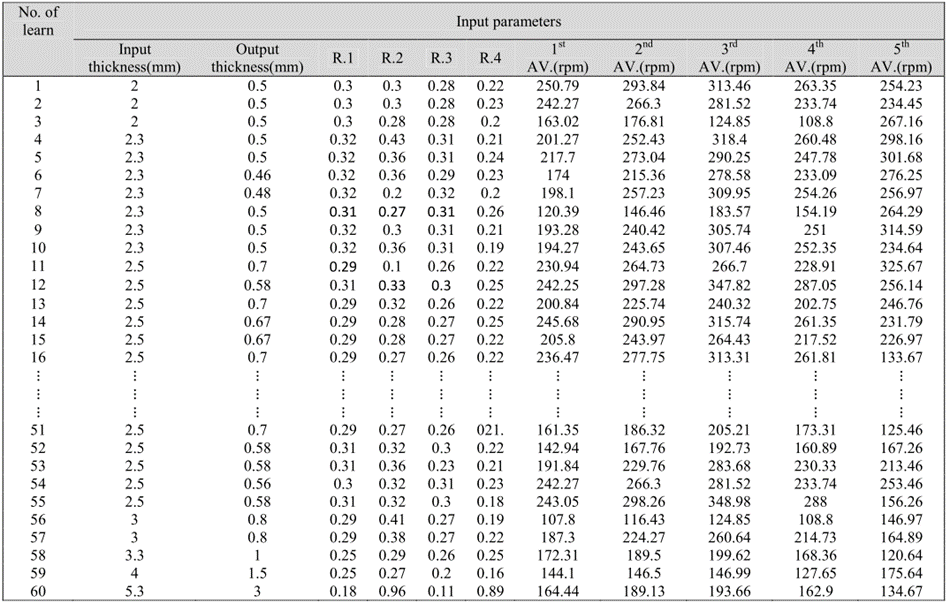Table 5. The applied input data for training the network corresponding to 2D simulation of tandem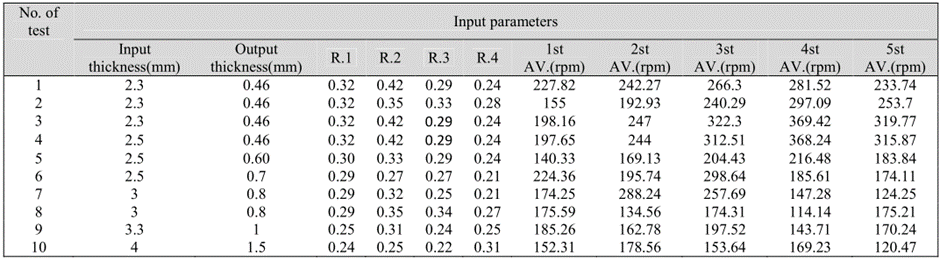Table 6. The applied input data for testing the network corresponding to 2D simulation of tandem cold

### 4. Results and Discussions

The developed ANN model is adopted to predict the inter-stand tensions in a tandem cold rolling mill. The performance of this proposed ANN model of training and testing data is plotted in Figures 7 and 8, respectively for all inter-stand tensions; therefore, the ANN appears to have a high generalization capability. The neural network model with eleven input neurons is trained with 60 training datasets and cross-validated (tested) through 10 datasets.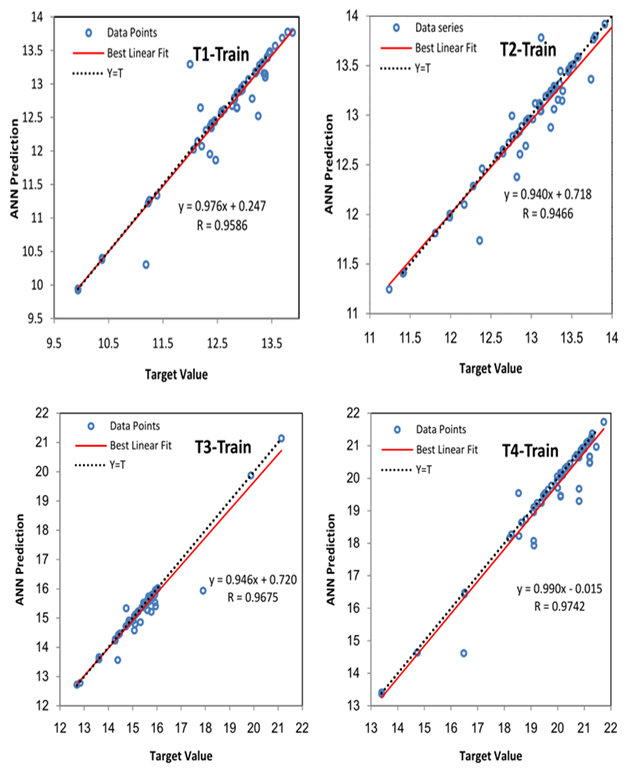Figure 7. The training performances of this proposed ANN model in prediction of inter-stand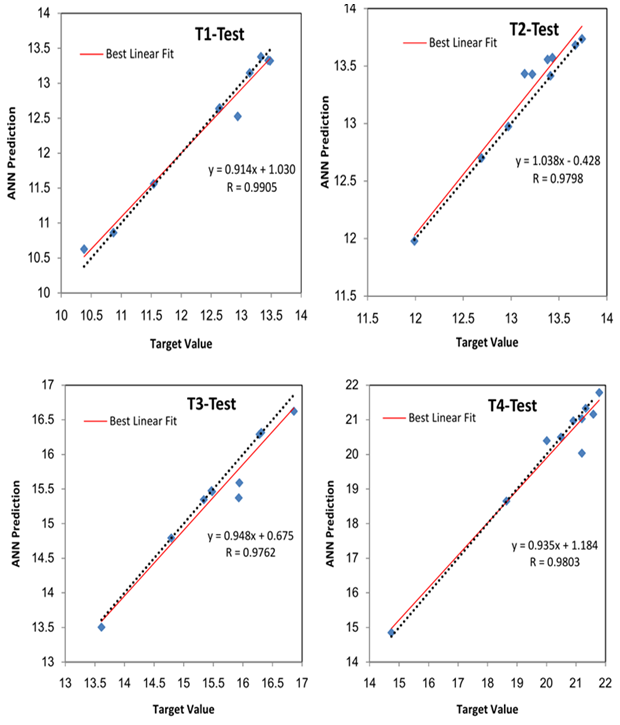Figure 8. The testing performance of the proposed ANN model in prediction of inter-stand
The testing and training performances are presented in Fig. 7 and 8 for proposed ANN model. All “T”s in two figures show all inter-stand tensions. This examination was performed for four levels as T1, T2, T3 and T4.
Here, the performance of the neural network modeling is evaluated through the mean absolute percentage error (MAPE), maximum percentage error, sum squared error (SSE), root mean-square error (RMSE) and the correlation coefficient (R). For the test sets, the deviations of the predicted outputs from the measured experimental data are determined to evaluate the accuracy of this proposed ANN models in predicting the desired inter-stand tensions. As mentioned, the MAPE is the computed average of the absolute percentage errors calculated by equation 2 :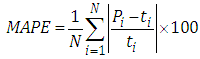(2)
In addition to this performance the predictability of the network is quantified in terms of average root mean square error (RMSE) expressed as: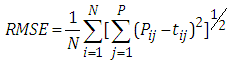(3)
The typical performance function which is defined by the equation bellow (4) is used for training a feed forward neural network which is the sum of squares of network errors, named SSE.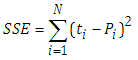(4)
Where, t is the target value and P is the predicted value obtained from the neural network model in these equations. N is the total number of data applied in the investigation and p is the number of variables in the output, equal to 4 in this study. It is obvious that SSE, RMSE and MAPE are involved with the relative error between experimental and predicted values. As seen in Table 7, a high coefficient of correlation and a low (MAPE) are obtained for the training and testing data sets for all inter-stand tensions. This proposed ANN model has the correlation coefficients of 0.9586, 0.9798, 0.9762 and 0.9742, respectively for training data sets and 0.9905, 0.9798, 0.9762 and 0.9803, respectively for the testing data sets (table 7). The (MAPE) of the inter-stand tensions are about 4.6005, 5.0511, 4.4398 and 5.8082% for the training, respectively for inter-stand tensions and the (MAPE) of the inter-stand tensions are about 2.3792, 5.2034, 1.3219 and 2.4758% for the testing sets. As it is seen these mean absolute percentage errors are reasonable. Furthermore, the models provided highly reasonable SSE of as low as 0.0820 and 0.0691, respectively for the training and testing sets of the inter-stand tensions.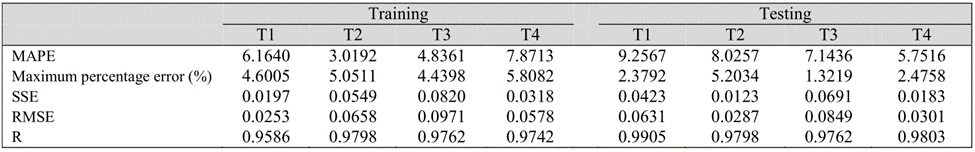Table 7. Statistical analysis of the performance of ANN model for training and testing predictions
The percentage error deviations of predicted inter-stand tensions through ANN from actual target results are illustrated in Figure 9. The ANN is capable of generalizing between input and output variables reasonably. The predictions are good not only for the training set, but also for the unseen testing set. All the predictions of the testing data fall within the 5.8% boundaries and within is less than 10% for the training data. It can be justified by Fig.9 which is related to “Relative Error %”. These percentages were calculated by training and testing data from Fig.9. It is sufficient to use the developed ANN models for the inter-stand tensions prediction, instead of the time consuming FE model. This quick response feature is a desirable property for online control and optimization, such as mill setup, dynamic adjustment and control. Hence, the resulted model here is quite suitable for online control of cold rolling mills in predicting appropriate rolling conditions due to the input parameters. The neural network model is essentially a complicated nonlinear model, consisting of numerous activation functions and interconnecting weights.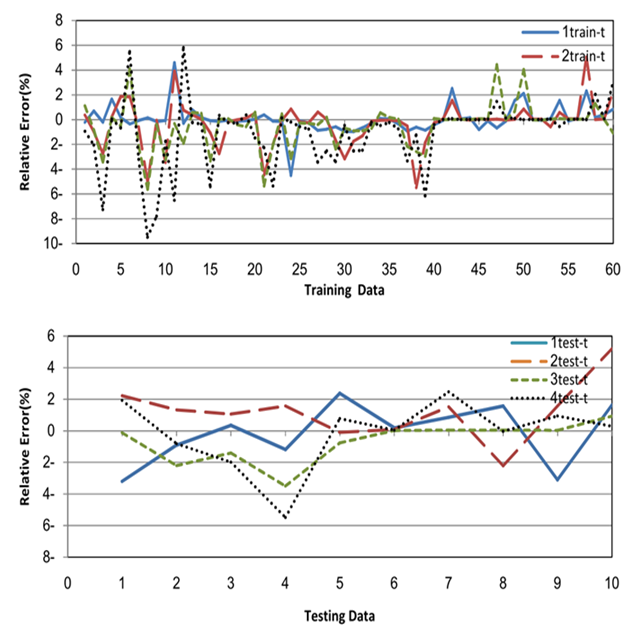Figure 9. Percentage error deviations of predicted inter-stand tensions from actual target results (a)
Figure 9 shows the percentage error deviations of predicted inter-stand tensions through ANN from actual target results. The resulted model in this figure is quite suitable for online control of cold rolling mills in predicting appropriate rolling conditions due to the input parameters. The neural network model is essential for a complicated non-linear model, consisting of numerous activation functions and interconnecting weights. In Fig.9, the Green line is 3train-t and the dotted line is 4train-t. 1train-t and 2train-t represent training sets for predictions. All predictions for training data are less than 10%.

### 5. Conclusions

In this article, an ANN model is developed for accurate prediction of inter-stand tensions in a tandem cold rolling mill with the objective to meet the quality standards of the final products. Through ABAQUS software, a 2D tandem cold rolling process of a strip is simulated and verified to provide a data basis for training, testing and validation of this neural network. The optimal configurations of the ANN model using BP algorithm are found to be 11-42-4 by trial and error. The optimum network architecture is obtained by evaluating the performance of ANNs with different number of hidden layers and neurons. By designing an algorithm of neural networks and training it through FE simulation results, the effect of parameters are investigated on the rolling process and the accuracy of the obtained results are predicted by ANN. The results indicate that this ANN model is a promising one in prediction of inter-stand tensions instead of time consuming experimental trials. The proposed neural network approach reduces the number and the cost of experimental tests commonly required in determining the inter-stand tensions. Adopting this ANN model is feasible for on-line control and rolling schedule optimization.